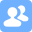# 1. Self-introduction

My name is Fuze Sun, a junior student at FZU. I like comic and animation, and I also like football. So far, my life is very peaceful and smoetimes boring, I really need a girlfriend to change this situation, Well, maybe it is too difficult for someone like me.

# 2. The skills I've learned and preferred

## 2.1 Programming language

After two and a half years of study, I've learned the basic knowledge of Java, c++, c, matlab and so on. It seems like I have learned a lot. Well, acturally, the hardest thing I can do is only to create a class by Java or c++. Programming is not the most important curse. I can not write a web page or APP. So software engineering may be the most difficult course for me in this term. Even though I will try my best to finish all the Lab perfectly.

## 2.2 Other courses I have

We also have other courses such as embedded system, signal&system and control system and so on. In the last term, my teammates and me build a robot which can automatically follow the ultrasonic signal. In my free time, I learned video clip. If you're interested, I'm very glad you to follow my bilibili account.

## 2.3 Skills I prefer

Nowadays, The concept of artificial intelligence is catching on. And I tried drawing with artificial intelligence. Hope one day, I can build an artificial intelligence project by myself.The picture I painted with artificial intelligence

# 3. The amount of code

The longest code I have written is about 180 lines. Here is an AVLtree class.

``````public class AVLtree {
public Node root;
public class Node{
int value;
Node left;
Node right;
Node parent;
public Node(int value){
this.value = value;
}
public Node(Node node,int value){
this.parent = node;
this.left = null;
this.right = null;
this.value = value;
}
}
public AVLtree() {
root = null;
}
public void insert(int key) {
Node n = new Node(key);
Node current = root;
Node p;
p = current;
if(root == null) {
root = n;
}
else {

while(true) {
p = current;
if(key < current.value) {
current = current.left;
if(current == null) {
Node n1 = new Node(p,key);
p.left = n1;
break;
}
}else{
current = current.right;
if(current == null) {
Node n1 = new Node(p,key);
p.right = n1;
break;
}
}
}
}
rebuild(p);
}
private Node rebuild(Node p) {
while(p != null){
if(getHight(p.left)-getHight(p.right) == 2){
if(getHight(p.left.left)-getHight(p.left.right) == 1)
rotateWithLeftChild(p);
else {
doubleWithLeftChildRightSubtree(p);
}
}else if(getHight(p.left)-getHight(p.right) == -2){
if(getHight(p.right.right)-getHight(p.right.left) == 1)
rotateWithRightChild(p);
else {
doubleWithRightChildLeftSubtree(p);
}
}
p = p.parent;

}
return p;
}
public int getHight(Node node){
if(node == null){
return 0;
}
return 1+Math.max(getHight(node.left),getHight(node.right));
}
private Node rotateWithLeftChild(Node k2) {
Node k1 = k2.left;
if(k2 != null) {
k2.left = k1.right;
if(k1.right != null) {
k1.right.parent = k2;
}
k1.parent = k2.parent;
if(k2.parent ==null) {
this.root = k1;
}
else if(k2.parent.right == k2) {
k2.parent.right = k1;
}
else if(k2.parent.left == k2) {
k2.parent.left = k1;
}
k1.right = k2;
k2.parent = k1;
}
return k1;
}
private Node rotateWithRightChild(Node k2) {
Node k1 = k2.right;
if(k2 != null) {
k2.right = k1.left;
if(k1.left != null) {
k1.left.parent = k2;
}
k1.parent = k2.parent;
if(k2.parent ==null) {
this.root = k1;
}
else if(k2.parent.right == k2) {
k2.parent.right = k1;
}
else if(k2.parent.left == k2) {
k2.parent.left = k1;
}
k1.left = k2;
k2.parent = k1;
}
return k1;
}
private Node doubleWithLeftChildRightSubtree(Node k3) {
k3.left = rotateWithRightChild(k3.left);
return rotateWithLeftChild(k3);
}
private Node doubleWithRightChildLeftSubtree(Node k3) {
k3.right = rotateWithLeftChild(k3.right);
return rotateWithRightChild(k3);
}
public void sortAscending(Node n) {
if(n!= null) {
sortAscending(n.left);
System.out.print(n.value + " ");
sortAscending(n.right);
}
}
public Node delete(Node root,int v) {
if(v<root.value) {
root.left = delete(root.left,v);
}
else if(v>root.value) {
root.right = delete(root.right,v);
}
else {
if(root.left!=null && root.right!=null) {
Node n = root.right;
while(n.left!=null) {
n=n.left;
}
root.value = n.value;
root.right = delete(root.right,n.value);
}
else {
root = (root.left!=null)?root.left:root.left;
}
}
if(root==null) {
return(root);
}
if(getHight(root.left)-getHight(root.right) == 2){
if(getHight(root.left.left)-getHight(root.left.right) == 1)
return(rotateWithLeftChild(root));
else {
return(doubleWithLeftChildRightSubtree(root));
}
}else if(getHight(root.left)-getHight(root.right) == -2){
if(getHight(root.right.right)-getHight(root.right.left) == 1)
return(rotateWithRightChild(root));
else {
doubleWithRightChildLeftSubtree(root);
}
}
return root;
}
}
``````

I hope we can finish a project with thousands of lines.

# 4. Course Planning

In this course, I want to have a better understanding of software engineering. As the team leader, I hope to lead our team to cooperate well and complete the tasks of this semester together. To ahieve this goal, I will try my best to learn relevant knowledge(database, Java...) and I will also assign appropriate tasks to my team members.

The Link of Requirement of This Assignmenthttps://bbs.csdn.net/topics/608734618
The Aim of This Assignmentintroduction
MU STU ID and FZU STU ID20124261 832002225
...全文
74 回复 打赏 收藏 转发到动态 举报05 group - topic selection and requirement analysis report
This is the blog of Group 05 for Lab3-1 (Final version).MU-SE: Course summary
Summary Blog for EE308FZ.

285发帖与我相关我的任务• 近7日
• 近30日
• 至今